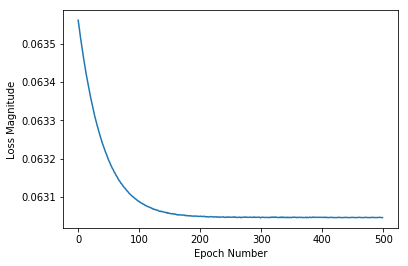## 公式

``````f = c * 1.8 + 32
``````

## import dependencies 导入依赖项

``````from __future__ import absolute_import, division, print_function, unicode_literals

import tensorflow as tf
import numpy as np
``````

``````import logging
logger = tf.get_logger() #返回tf的日志实例
logger.setLevel(logging.ERROR)
``````

## Set up training data 建立训练数据

``````celsius_q = np.array([-40, -10, 0, 8, 15, 22, 38], dtype=float)
fahrenheit_a = np.array([-40, 14, 32, 46, 59, 72, 100], dtype=float)

for i,c in enumerate(celsius_q):
print("{} degrees Celsius = {} degrees Fahrenheit".format(c, fahrenheit_a[i]))
``````

``````-40.0 degrees Celsius = -40.0 degrees Fahrenheit
-10.0 degrees Celsius = 14.0 degrees Fahrenheit
0.0 degrees Celsius = 32.0 degrees Fahrenheit
8.0 degrees Celsius = 46.0 degrees Fahrenheit
15.0 degrees Celsius = 59.0 degrees Fahrenheit
22.0 degrees Celsius = 72.0 degrees Fahrenheit
38.0 degrees Celsius = 100.0 degrees Fahrenheit
``````

### 一些机器学习术语：

• Feature（特征）：模型的输入。在这里即摄氏度
• Labels（标签）：模型的输出。在这里即华氏度
• Example（样本）：训练期间数据集的一行内容，可以是标注样本(labeled example)和无标注样本(unlabeled example)。在这里即一对摄氏度与华氏度数据，为标注样本

## Create the model 创建模型

### Build a layer 建立一个层

#### `tf.keras.layers.Dense`

• `units=1` 指定本层神经元数量。神经元的数量定义了本层需要有多少内部变量来学习解决这个问题。由于这是本模型的最后一层，因此它也代表模型输出的大小。（在多层神经网络中，该层的大小与形状需要与下一层的`input_shape`相匹配

• `input_shape=` 指定本层输入值为单值。表明这是一个包含单个成员的一维数组。由于这是本模型的第一层（也是唯一一层），因此该输入形状也是整个模型的输入形状。单值是浮点数，即摄氏度

``````l0 = tf.keras.layers.Dense(units=1, input_shape=)
``````

### Assemble layers into the model 将Layer组装到模型中

``````model = tf.keras.Sequential([l0])
``````

``````model = tf.keras.Sequential([ tf.keras.layers.Dense(units=1, input_shape=) ])
``````

## Compile the model, with loss and optimizer functions 使用损失和优化器功能编译模型

• `Loss function` 一种衡量结果与预期相差多少的方法（测得的差异称为`loss`(损失)）

• `Optimizer function` 一种调整内部值以减少损耗的方法

• `Metrics function` （较复杂，此处不用，日后再看）

``````model.compile(loss='', optimizer=tf.keras.optimizers.Adam(0.1))
``````

## Train the model 训练模型

``````history = model.fit(celsius_q, fahrenheit_a, epochs=500, verbose=False) #fit方法返回History对象
print("Finished training the model")
``````

``````Finished training the model
``````

verbose参数

• 默认为1
• verbose = 0 为不在标准输出流输出日志信息
• verbose = 1 为输出进度条记录
• verbose = 2 为每个epoch输出一行记录

History对象

## Display training statistics 展示训练统计数据

``````import matplotlib.pyplot as plt
plt.xlabel('Epoch Number') #x坐标轴
plt.ylabel("Loss Magnitude") #y坐标轴
plt.plot(history.history['loss']) #标点
plt.show()
``````## Use the model to predict values 使用模型预测数值

``````print(model.predict([100.0])) #向模型输入100.0
``````

``````[[211.74744]]
``````

``````100*1.8 + 32 = 212
``````

## To review 回顾

1. 我们创建了一个含有单层神经元的模型
2. 将模型训练了3500次（7对数据，500轮）

``````l0 = tf.keras.layers.Dense(units=1, input_shape=)
model = tf.keras.Sequential([l0])
history = model.fit(celsius_q, fahrenheit_a, epochs=500, verbose=False)
model.predict([100.0])
``````

## Looking at the layer weights 查看Layer权重

``````print("These are the layer variables: {}".format(l0.get_weights())) #以包含Numpy矩阵的列表形式返回层的权重
``````

``````These are the layer variables: [array([[1.7979496]], dtype=float32), array([31.952478], dtype=float32)]
``````

### A little experiment 小实验

``````l0 = tf.keras.layers.Dense(units=4, input_shape=)
l1 = tf.keras.layers.Dense(units=4)
l2 = tf.keras.layers.Dense(units=1)
model = tf.keras.Sequential([l0, l1, l2])
model.fit(celsius_q, fahrenheit_a, epochs=500, verbose=False)
print("Finished training the model")
print("Model predicts that 100 degrees Celsius is: {} degrees Fahrenheit".format(model.predict([100.0])))
print("These are the l0 variables: {}".format(l0.get_weights()))
print("These are the l1 variables: {}".format(l1.get_weights()))
print("These are the l2 variables: {}".format(l2.get_weights()))
``````

``````Finished training the model
Model predicts that 100 degrees Celsius is: [[211.74742]] degrees Fahrenheit #得到预测结果仍然与实际相近
These are the l0 variables: [array([[-0.52520204, -0.25983605,  0.27832836,  0.11471745]],
dtype=float32), array([-3.468158 , -3.3907545,  3.5017395,  2.1893277], dtype=float32)]
These are the l1 variables: [array([[-0.37798777,  0.6455525 ,  0.7230809 ,  0.3954812 ],
[-0.85110897,  0.6376249 ,  0.31107017, -0.70929325],
[-0.534542  , -0.980425  , -0.92369473,  0.72322994],
[ 0.38675487,  0.41835558, -0.24832523, -0.08700816]],
dtype=float32), array([ 3.2148993, -3.1253922, -3.340591 ,  3.433869 ], dtype=float32)]
These are the l2 variables: [array([[ 0.74975073],
[-0.56715256],
[-1.4492323 ],
[ 0.39553005]], dtype=float32), array([3.2948794], dtype=float32)]
``````